### 14.6.3.1 Expressing systems in terms of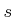,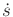, and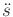Suppose that a system is given in the form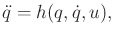(14.31)

in which there areaction variables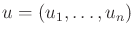. It may be helpful to glance ahead to Example 14.6, which will illustrate the coming concepts for the simple case of double integrators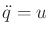. The acceleration inis determined from the state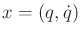and action. Assume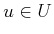, in which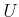is an-dimensional subset of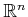. If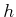is nonsingular at, then an-dimensional set of possible accelerations arises from choices of. This means it is fully actuated. If there were fewer thanaction variables, then there would generally not be enough freedom to follow a specified path. Therefore,must be-dimensional. Which choices of, however, constrain the motion to follow the given path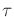? To determine this, the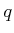,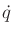, and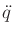variables need to be related to the path domainand its first and second time derivativesand, respectively. This leads to a subset ofthat corresponds to actions that follow the path.

Suppose that,,, and a pathare given. The configuration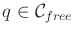is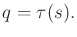(14.32)

Assume that all first and second derivatives ofexist. The velocitycan be determined by the chain rule as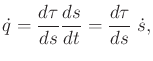(14.33)

in which the derivative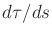is evaluated at. The acceleration is obtained by taking another derivative, which yields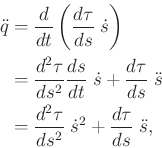(14.34)

by application of the product rule. The full statecan be recovered from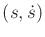using (14.32) and (14.33).

The next step is to obtain an equation that looks similar to (14.31), but is expressed in terms of,, and. A function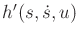can be obtained from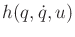by substituting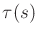forand the right side of (14.33) for: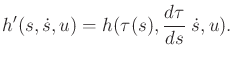(14.35)

This yields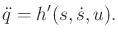(14.36)

For a given state(which can be obtained fromand), the set of accelerations that can be obtained by a choice ofin (14.36) is the same as that for the original system in (14.31). The only difference is thatis now constrained to a 2D subset of, which are the states that can be reached by selecting values forand.

Applying (14.34) to the left side of (14.36) constrains the accelerations to cause motions that follow. This yields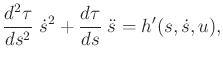(14.37)

which can also be expressed as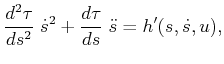(14.38)

by moving the first term of (14.34) to the right. Note thatequations are actually represented in (14.38). For eachin which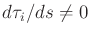, a constraint of the form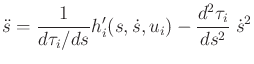(14.39)

is obtained by solving for.

Steven M LaValle 2020-08-14